A-A+

# [Tkinter Gui 教程12] 布局管理 (Pack Place Grid)

2019年12月20日 11:29 阅读 529 views 次

## 简介:

• pack
• grid
• place

• 将控件放置在屏幕上,包括控件的位置及控件的大小
• 将控件注册到本地窗口系统中
• 管理控件在屏幕上的显示

## Pack 布局管理

pack 是三种布局管理中最常用的. 另外两种布局需要精确指定控件具体的显示位置, 而 pack 布局可以指定相对位置, 精确的位置会由 pack 系统自动完成. 这也使得 pack 布局没有另外两种布局方式灵活. 所以 pack 是简单应用的首选布局, 比如只是想要将控件水平或垂直的逐个放在一起:

``````from Tkinter import *

root = Tk()
Label(root, text="Red Sun", bg="red", fg="white").pack()
Label(root, text="Green Grass", bg="green", fg="black").pack()
Label(root, text="Blue Sky", bg="blue", fg="white").pack()
mainloop()``````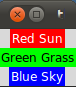### fill 控件填充方式

``````from Tkinter import *

root = Tk()
w = Label(root, text="Red Sun", bg="red", fg="white")
w.pack(fill=X)
w = Label(root, text="Green Grass", bg="green", fg="black")
w.pack(fill=X)
w = Label(root, text="Blue Sky", bg="blue", fg="white")
w.pack(fill=X)
mainloop()``````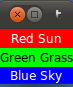Pack 可以在四个方面控制控件边距: 内边距, 外边距, 水平边距, 垂直边距:

`padx` - 设置水平方向的外边距

``````from Tkinter import *

root = Tk()
w = Label(root, text="Red Sun", bg="red", fg="white")
w = Label(root, text="Green Grass", bg="green", fg="black")
w = Label(root, text="Blue Sky", bg="blue", fg="white")
mainloop()``````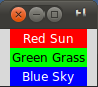`pady` - 设置竖直方向的外边距

``````from Tkinter import *

root = Tk()
w = Label(root, text="Red Sun", bg="red", fg="white")
w = Label(root, text="Green Grass", bg="green", fg="black")
w = Label(root, text="Blue Sky", bg="blue", fg="white")
mainloop()``````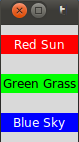`ipadx` - 设置水平方向的内边距

``````from Tkinter import *

root = Tk()
w = Label(root, text="Red Sun", bg="red", fg="white")
w.pack()
w = Label(root, text="Green Grass", bg="green", fg="black")
w = Label(root, text="Blue Sky", bg="blue", fg="white")
w.pack()
mainloop()``````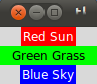`ipady` - 设置竖直方向的内边距

``````from Tkinter import *

root = Tk()
w = Label(root, text="Red Sun", bg="red", fg="white")
w.pack()
w = Label(root, text="Green Grass", bg="green", fg="black")
w = Label(root, text="Blue Sky", bg="blue", fg="white")
mainloop()``````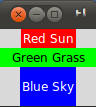### side 顺次放置控件

``````from Tkinter import *

root = Tk()
w = Label(root, text="red", bg="red", fg="white")
w = Label(root, text="green", bg="green", fg="black")
w = Label(root, text="blue", bg="blue", fg="white")
mainloop()``````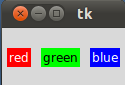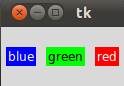## Place 布局管理

Place 布局管理可以显式的指定控件的绝对位置或相对于其他控件的位置. 要使用 Place 布局, 调用相应控件的 `place()` 方法就可以了. 所有 tkinter 的标准控件都可以调用 `place()` 方法.

``````import Tkinter as tk
import random

root = tk.Tk()
# width x height + x_offset + y_offset:
root.geometry("170x200+30+30")

languages = ['Python','Perl','C++','Java','Tcl/Tk']
labels = range(5)
for i in range(5):
ct = [random.randrange(256) for x in range(3)]
brightness = int(round(0.299*ct + 0.587*ct + 0.114*ct))
ct_hex = "%02x%02x%02x" % tuple(ct)
bg_colour = '#' + "".join(ct_hex)
l = tk.Label(root,
text=languages[i],
fg='White' if brightness < 120 else 'Black',
bg=bg_colour)
l.place(x = 20, y = 30 + i*30, width=120, height=25)

root.mainloop()``````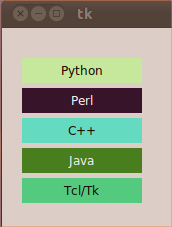## Grid 布局管理

Pack 作为首选的布局管理方式, 其运作方式并不是特别易于理解. 已经由 Pack 布局完成的设计也很难做出改变. Grid 布局在1996年作为另一种可供选择的布局方式被引入. Grid 布局方式易学易用, 但似乎大家还是习惯用 Pack.

Grid 在很多场景下是最好用的布局方式. 相比而言, Pack 布局在控制细节方面有些力不从心. Place 布局虽然可以完全控制控件位置, 但这也导致使用 Place 会比其他两种布局方式更加复杂.

Grid 把控件位置作为一个二维表结构来维护, 即按照行列的方式排列控件: 控件位置由其所在的行号和列号决定. 行号相同而列号不同的几个控件会被彼此上下排列; 列号相同而行号不同的几个控件会被彼此左右排列.

``````from Tkinter import *

colours = ['red','green','orange','white','yellow','blue']

r = 0
for c in colours:
Label(text=c, relief=RIDGE,width=15).grid(row=r,column=0)
Entry(bg=c, relief=SUNKEN,width=10).grid(row=r,column=1)
r = r + 1

mainloop()``````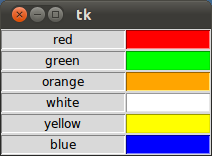## 相关tkinter 教程系列

### 布施恩德可便相知重

#### 微信扫一扫打赏#### 支付宝扫一扫打赏×